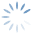Help OnLine | Options Strategy – Standard Deviations
3613

# Options Strategy – Standard Deviations

Click on the image to expand

It is the dispersion of the individual observations around the arithmetic mean and is used to evaluate the deviation from the so-called “balance”.

The standard deviation that is indicated with the Greek letter “Sigma”, indicates how much each value moves away from the arithmetic mean of the values and is the weighted average of the deviations, of the deviations, from the arithmetic mean.
Values then squared up.
In statistics it is also called “square root of variance” or “standard quadratic gap”. It is the first statistical analysis tool to determine if a set of values deviates from the so-called equilibrium and by how much. And that is from a quantitative indication on how much the distribution of the data series has been “regular”.

In beeTrader  the value of the standard deviation is calculated in a period of 21 days of the open stock exchange and then, drawn the values in the payoff chart with two vertical green lines placed at a number of deviations equal to those set by the user. By default they are represented at 2 Std. Dev.
What you want to display is the distance between the lines of the Std. Dev. and the Last of the underlying in such a way as to have a statistical area that is likely not to be crossed at the time of the observation.

Probability equivalent to the deviations set:

• 1 Std. Dev. equals 68%
• 2 Std. Dev. equivalent to 95%